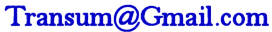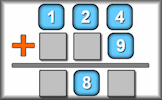# Algebraic Fractions

## A mixture of algebraic fraction calculations and simplifications.

##### Level 1Level 2Level 3Level 4Level 5Exam-StyleDescriptionHelpMore Algebra

This is level 3; Adding and subtracting two algebraic fractions. You can earn a trophy if you get at least 9 correct. Give your answers as fractions in their lowest terms. Do not factorise and type letters in alphabetical order where appropriate.

$$\frac{a}{3}+\frac{b}{12}$$

 =$$\frac{c}{3}+\frac{d}{4}$$

 =$$\frac{e}{5}-\frac{f}{7}$$

 =$$\frac{3g}{4}-\frac{4h}{5}$$

 =$$\frac{7j}{8}+\frac{7k}{8}$$

 =$$\frac{3}{m}+\frac{5}{n}$$

 =$$\frac{3}{4p}+\frac{6}{7q}$$

 =$$\frac{5}{6r}+\frac{10}{11s}$$

 =$$\frac{3t}{4u}-\frac{4}{5v}$$

 =$$\frac{5w}{6y}-\frac{8x}{9z}$$

 =$$\frac{x}{6}+\frac{x}{3}+\frac{x}{4}$$

 =$$\frac{1}{x}+\frac{1}{2x}-\frac{1}{3x}$$

 =Check

This is Algebraic Fractions level 3. You can also try:
Level 1 Level 2 Level 4 Level 5

## Instructions

Try your best to answer the questions above. Type your answers into the boxes provided leaving no spaces. As you work through the exercise regularly click the "check" button. If you have any wrong answers, do your best to do corrections but if there is anything you don't understand, please ask your teacher for help.

When you have got all of the questions correct you may want to print out this page and paste it into your exercise book. If you keep your work in an ePortfolio you could take a screen shot of your answers and paste that into your Maths file.

## Transum.org

This web site contains over a thousand free mathematical activities for teachers and pupils. Click here to go to the main page which links to all of the resources available.

Please contact me if you have any suggestions or questions.## More Activities:

Mathematicians are not the people who find Maths easy; they are the people who enjoy how mystifying, puzzling and hard it is. Are you a mathematician?

Comment recorded on the 5 April 'Starter of the Day' page by Mr Stoner, St George's College of Technology:

"This resource has made a great deal of difference to the standard of starters for all of our lessons. Thank you for being so creative and imaginative."

Comment recorded on the 9 May 'Starter of the Day' page by Liz, Kuwait:

"I would like to thank you for the excellent resources which I used every day. My students would often turn up early to tackle the starter of the day as there were stamps for the first 5 finishers. We also had a lot of fun with the fun maths. All in all your resources provoked discussion and the students had a lot of fun."

#### Nine DigitsArrange the given digits one to nine to make three numbers such that two of them add up to the third. This is a great puzzle for practicing standard pen and paper methods of three digit number addition and subtraction.

There are answers to this exercise but they are available in this space to teachers, tutors and parents who have logged in to their Transum subscription on this computer.

A Transum subscription unlocks the answers to the online exercises, quizzes and puzzles. It also provides the teacher with access to quality external links on each of the Transum Topic pages and the facility to add to the collection themselves.

Subscribers can manage class lists, lesson plans and assessment data in the Class Admin application and have access to reports of the Transum Trophies earned by class members.

If you would like to enjoy ad-free access to the thousands of Transum resources, receive our monthly newsletter, unlock the printable worksheets and see our Maths Lesson Finishers then sign up for a subscription now:

Subscribe

## Go Maths

Learning and understanding Mathematics, at every level, requires learner engagement. Mathematics is not a spectator sport. Sometimes traditional teaching fails to actively involve students. One way to address the problem is through the use of interactive activities and this web site provides many of those. The Go Maths page is an alphabetical list of free activities designed for students in Secondary/High school.

## Maths Map

Are you looking for something specific? An exercise to supplement the topic you are studying at school at the moment perhaps. Navigate using our Maths Map to find exercises, puzzles and Maths lesson starters grouped by topic.

## Teachers

If you found this activity useful don't forget to record it in your scheme of work or learning management system. The short URL, ready to be copied and pasted, is as follows:

Do you have any comments? It is always useful to receive feedback and helps make this free resource even more useful for those learning Mathematics anywhere in the world. Click here to enter your comments.For All:

## Description of Levels

CloseLevel 1 - Simplifying algebraic fractions.

Level 2 - Multiplying and dividing algebraic fractions.

Level 3 - Adding and subtracting two algebraic fractions.

Level 4 - Simplifying algebraic fractions by factorising.

Level 5 - Mixed algebraic fraction questions.

Exam Style questions are in the style of GCSE or IB/A-level exam paper questions and worked solutions are available for Transum subscribers.

More on this topic including lesson Starters, visual aids and investigations.

Answers to this exercise are available lower down this page when you are logged in to your Transum account. If you don’t yet have a Transum subscription one can be very quickly set up if you are a teacher, tutor or parent.

## Curriculum Reference

See the National Curriculum page for links to related online activities and resources.

## Example

Don't wait until you have finished the exercise before you click on the 'Check' button. Click it often as you work through the questions to see if you are answering them correctly. You can double-click the 'Check' button to make it float at the bottom of your screen.

Answers to this exercise are available lower down this page when you are logged in to your Transum account. If you don’t yet have a Transum subscription one can be very quickly set up if you are a teacher, tutor or parent.

Close